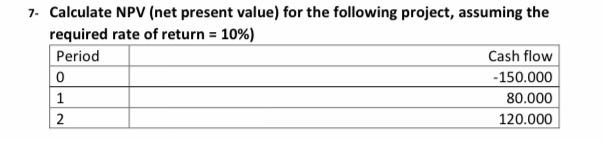### Create an Account

Already have account?

### Forgot Your Password ?

Home / Questions / Calculate NPV (net present value) for the following project, assuming the required rate of...

# Calculate NPV (net present value) for the following project, assuming the required rate of return = 10%) Period Cash flow 0 - 150.000 1 80.000 2 120.000

Calculate NPV (net present value) for the following project, assuming the required rate of return = 10%) Period Cash flow 0 - 150.000 1 80.000 2 120.000Apr 15 2021 View more View Less

#### Answer (Solved)Subscribe To Get Solution